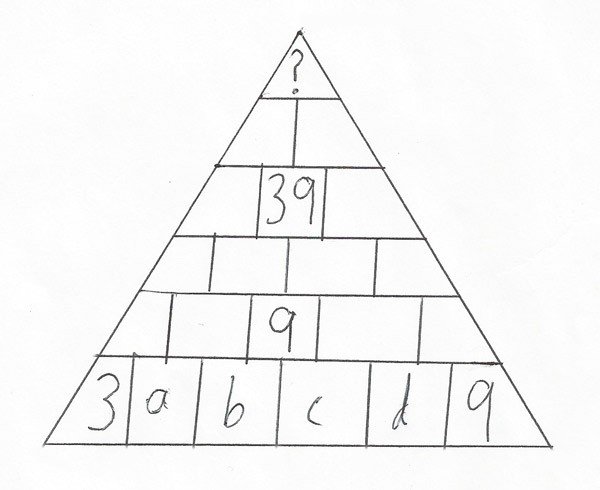Home > Puzzles of the month

# December 2014 - Pyramid of numbers (Solution)

The answer is 162. There are many ways to do this. Here is one solution. Call the four numbers in the bottom row a, b, c, and d.We now have 9 = b+c. Also, 39 = a+3b+3c+d. Now, the top number is 3+5a+10b+10c+5d+9 = 5(a+2b+2c+d)+12 = 5(a+3b+3c+d-b-c)+12 = 5(39-9)+12 = 5(30)+12 = 150+12 = 162.

This algebraic approach works. However, a trial-and-error approach is also possible. Suppose you guessed a number. This would let you work down a bit until you needed to guess another number. This lets you figure out the top number, which will always be 162.

One remarkable fact about this puzzle is that while the top number is always 162, all the other numbers apart from the given numbers can be any number at all! This strongly contrasts with the traditional pyramid of numbers puzzle where the entire bottom row is given, forcing all other numbers to have a specific value. Here, I made a unique solution using the least amount of information possible. If I removed a number, it would be impossible. If all numbers were at the bottom, removing a number would still make it impossible. But there 6 numbers are used, whereas here only 4 numbers are necessary. This puzzle is possible, but requires more than just addition. It also has nothing redundant whatsoever.

•The puzzle

•Hall of Fame